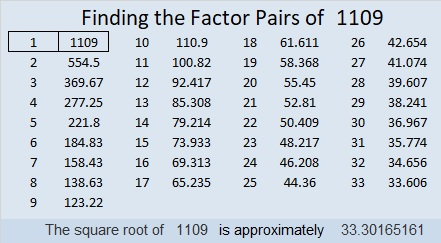# 1109 Mystery Level

Is this puzzle easy to solve or difficult? That’s part of the mystery. I hope you will give it a try and figure it out.Print the puzzles or type the solution in this excel file: 10-factors-1102-1110

Here is some information about the number 1109:

• 1109 is a prime number.
• Prime factorization: 1109 is prime.
• The exponent of prime number 1109 is 1. Adding 1 to that exponent we get (1 + 1) = 2. Therefore 1109 has exactly 2 factors.
• Factors of 1109: 1, 1109
• Factor pairs: 1109 = 1 × 1109
• 1109 has no square factors that allow its square root to be simplified. √1109 ≈ 33.30165

How do we know that 1109 is a prime number? If 1109 were not a prime number, then it would be divisible by at least one prime number less than or equal to √1109 ≈ 33.3. Since 1109 cannot be divided evenly by 2, 3, 5, 7, 11, 13, 17, 19, 23, 29 or 31, we know that 1109 is a prime number.25² + 22² = 1109

1109 is the hypotenuse of a Pythagorean triple:
141-1100-1109 calculated from 25² – 22², 2(25)(22), 25² + 22²

Here’s another way we know that 1109 is a prime number: Since its last two digits divided by 4 leave a remainder of 1, and 25² + 22² = 1109 with 25 and 22 having no common prime factors, 1109 will be prime unless it is divisible by a prime number Pythagorean triple hypotenuse less than or equal to √1109 ≈ 33.3. Since 1109 is not divisible by 5, 13, 17, or 29, we know that 1109 is a prime number.

1109 is palindrome 919 in BASE 11 because 9(121) + 1(11) + 9(1) = 1109

This site uses Akismet to reduce spam. Learn how your comment data is processed.